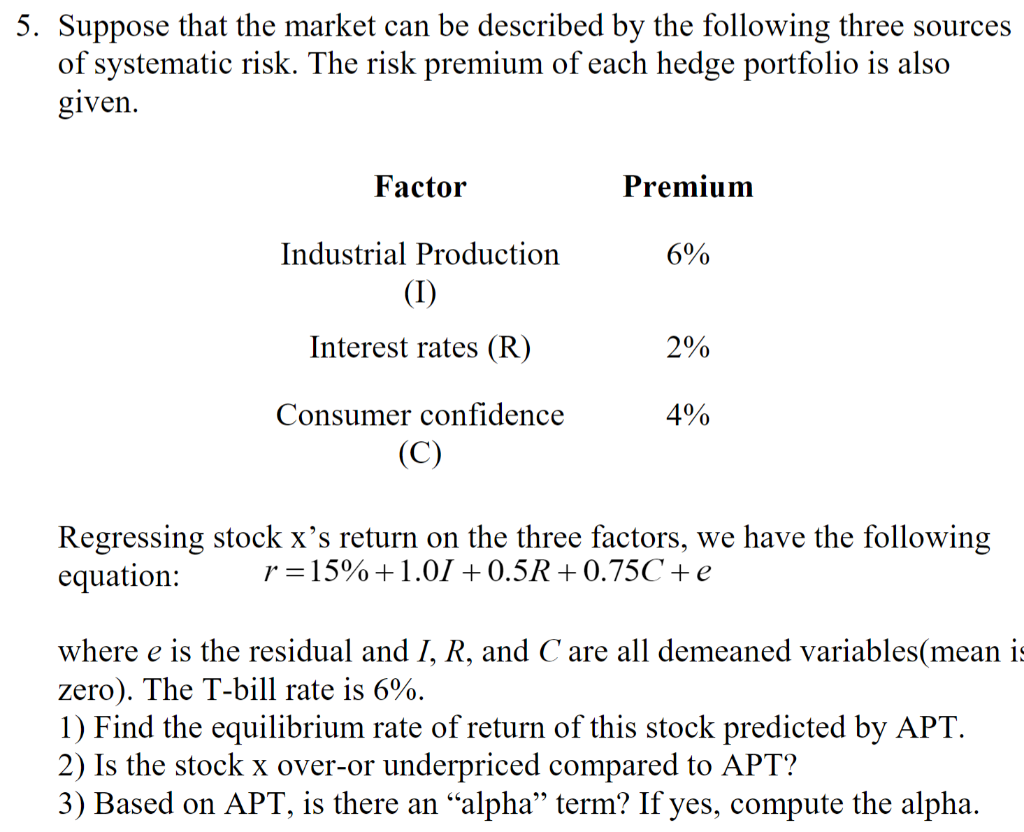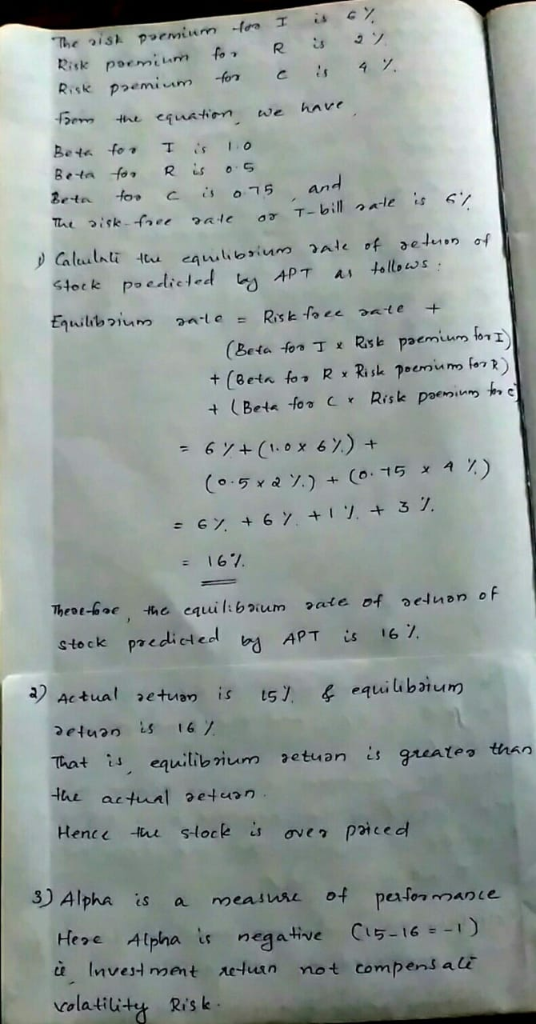Homework Help Question & Answers

# 5. Suppose that the market can be described by the following three sources of svstematic risk. Th...5. Suppose that the market can be described by the following three sources of svstematic risk. The risk premium of each hedge portfolio is also given Factor Premium Industrial Production 0 0 Interest rates (R) 0 Consumer confidence 4% Regressing stock x's return on the three factors, we have the following equation r 15% + l.OJ + 0.5R + 0.75C + e where e is the residual and I, R, and C' are all demeaned variables(mean i zero). The T-bill rate is 6%. 1) Find the equilibrium rate of return of this stock predicted by APT. 2) Is the stock x over-or underpriced compared to APT? 3) Based on APT, is there an "alpha" term? If yes, compute the alpha.

#### Homework Answers

ReportAnswer #1Add Homework Help Answer
##### Add Answer of: 5. Suppose that the market can be described by the following three sources of svstematic risk. Th...
Your Answer: Your Name: What's your source?
Not the answer you're looking for? Ask your own homework help question. Our experts will answer your question WITHIN MINUTES for Free.
More Homework Help Questions Additional questions in this topic.

• #### out of the four economic market models : competitive market, monopoly market, monopolistic competition and oligopolywhich is the best market model and which is the worst

Need Online Homework Help?

Get FREE EXPERT Answers
WITHIN MINUTES
Related Questions